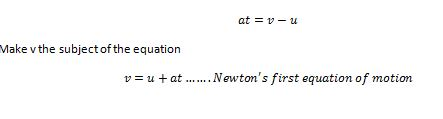Physic Tutorials

# Physics Formulas Kinematics With Graphs

Physics Formulas Kinematics With Appropriate Graphs

Kinematics in physics is about the motion of a body or an obeject. And the terms associated with kinematics are displacement, speed, velocity, and acceleration. These are the variables physicists play around in this topic.

Kinematics formulas

The starting point is from the definition of acceleration which is the rate of change of velocity. This is like a car travelling at a certain speed then the driver decided to move faster or slower by pressing the accelerator. The speedometer in the car will change and at that instance the car has either accelerate or decelerate.

Acceleration = change of velocity / time = (v – u) / t

u is the initial velocity, v is the final velocity, t is the time taken, and a is the acceleration

cross multiply,

Make v the subject of the equationThe first formula above is the Newton’s first equation of motion

The next step,

From average velocity,

Average velocity = s / t

Average velocity = (v +u)/2

Equate the two equations together, you get

s / t = (v +u)/2

Cross multiply and rearrange

s = [(v + u)t]/2

substitute v = u + at into  s = [(v + u)t]/2

you haveThis is the Newton’s second equation of motion

Recommended: Short notes on motion

Final step:

Make t the subject of the Newton’s first equation of motion

t = (v – u) / a

substitute t = (v – u) / a into Newton’s second equation of motion

Cross multiply,This is the Newton’s third equation of motion

The next thing is to look at Kinematics graphs### Bolarinwa Olajire

A lecturer, Educationist, PhD student at FUNAAB, and a Blogger.